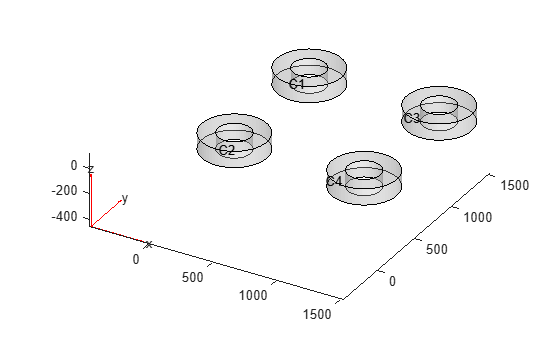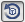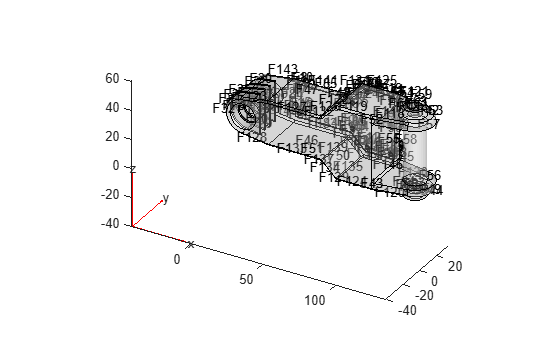# STL File Import

This example shows how to import a geometry from an STL file, and then plot the geometry. Generally, you create the STL file by exporting from a CAD system, such as SolidWorks®. For best results, export a fine (not coarse) STL file in binary (not ASCII) format. After importing, view the geometry using the `pdegplot` function. To see the face IDs, set the `FaceLabels` name-value pair to "`on"`.

View the geometry examples included with Partial Differential Equation Toolbox™.

```figure gm = fegeometry("Torus.stl"); pdegplot(gm)``````figure gm = fegeometry("Block.stl"); pdegplot(gm,FaceLabels="on",FaceAlpha=0.3)``````figure gm = fegeometry("Plate10x10x1.stl"); pdegplot(gm,FaceLabels="on",FaceAlpha=0.3)``````figure gm = fegeometry("Tetrahedron.stl"); pdegplot(gm,FaceLabels="on",FaceAlpha=0.3)``````figure gm = fegeometry("BracketWithHole.stl"); pdegplot(gm,FaceLabels="on",FaceAlpha=0.3)``````figure gm = fegeometry("DampingMounts.stl"); pdegplot(gm,CellLabels="on",FaceAlpha=0.3)``````figure gm = fegeometry("MotherboardFragment1.stl"); pdegplot(gm)``````figure gm = fegeometry("PlateHoleSolid.stl"); pdegplot(gm,FaceLabels="on",FaceAlpha=0.3)``````figure gm = fegeometry("PlateSquareHoleSolid.stl"); pdegplot(gm)``````figure gm = fegeometry("SquareBeam.stl"); pdegplot(gm,FaceLabels="on",FaceAlpha=0.3)``````figure gm = fegeometry("BracketTwoHoles.stl"); pdegplot(gm,FaceLabels="on",FaceAlpha=0.3)```To see hidden portions of the geometry, rotate the figure using Rotate 3D buttonor the `view` function. You can rotate the angle bracket to obtain the following view.

```figure pdegplot(gm,FaceLabels="on",FaceAlpha=0.3) view([-24 -19])``````figure gm = fegeometry("ForearmLink.stl"); pdegplot(gm,FaceLabels="on",FaceAlpha=0.3);```When you import a planar STL geometry, the toolbox converts it to a 2-D geometry by mapping it to the X-Y plane.

```figure gm = fegeometry("PlateHolePlanar.stl"); pdegplot(gm,"EdgeLabels","on")``````figure gm = fegeometry("PlateSquareHolePlanar.stl"); pdegplot(gm);```